GeeksforGeeks App
Open AppBrowser
Continue

## Related Articles

• Write an Interview Experience
• NumPy Tutorial

# How to access different rows of a multidimensional NumPy array?

Let us see how to access different rows of a multidimensional array in NumPy. Sometimes we need to access different rows of multidimensional NumPy array-like first row, the last two rows, and even the middle two rows, etc. In NumPy , it is very easy to access any rows of a multidimensional array. All we need to do is Slicing the array according to the given conditions. Whenever we need to perform analysis, slicing plays an important role.

Case 1: In 2-Dimensional arrays

Example 1: Accessing the First and Last row of a 2-D NumPy array

## Python3

 `# Importing Numpy module``import` `numpy as np`` ` `# Creating a 3X3 2-D Numpy array``arr ``=` `np.array([[``10``, ``20``, ``30``], ``                ``[``40``, ``5``, ``66``], ``                ``[``70``, ``88``, ``94``]])`` ` `print``(``"Given Array :"``)``print``(arr)`` ` `# Access the First and Last rows of array``res_arr ``=` `arr[[``0``,``2``]]``print``(``"\nAccessed Rows :"``)``print``(res_arr)`

Output: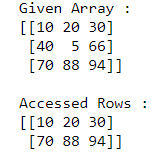In the above example, we access and print the First and Last rows of the 3X3 NumPy array.

Example 2: Accessing the Middle row of 2-D NumPy array

## Python3

 `# Importing Numpy module``import` `numpy as np`` ` `# Creating a 3X4 2-D Numpy array``arr ``=` `np.array([[``101``, ``20``, ``3``, ``10``], ``                ``[``40``, ``5``, ``66``, ``7``], ``                ``[``70``, ``88``, ``9``, ``141``]])``                ` `print``(``"Given Array :"``)``print``(arr)`` ` `# Access the Middle row of array``res_arr ``=` `arr[``1``]``print``(``"\nAccessed Row :"``)``print``(res_arr)`

Output: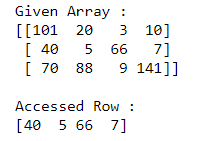In the above example, we access and print the Middle row of the 3X4 NumPy array.

Example 3: Accessing the Last three rows of 2-D NuNumPy py array

## Python3

 `# Importing Numpy module``import` `numpy as np`` ` `# Creating a 4X4 2-D Numpy array``arr ``=` `np.array([[``1``, ``20``, ``3``, ``1``], ``                ``[``40``, ``5``, ``66``, ``7``], ``                ``[``70``, ``88``, ``9``, ``11``],``               ``[``80``, ``100``, ``50``, ``77``]])`` ` `print``(``"Given Array :"``)``print``(arr)`` ` `# Access the Last three rows of array``res_arr ``=` `arr[[``1``,``2``,``3``]]``print``(``"\nAccessed Rows :"``)``print``(res_arr)`

Output: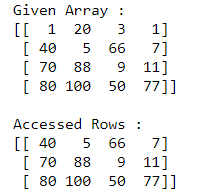In the above example, we access and print the last three rows of the 4X4 NumPy array.

Example 4: Accessing the First two rows of a 2-D NumPy array

## Python3

 `# Importing Numpy module``import` `numpy as np`` ` `# Creating a 5X4 2-D Numpy array``arr ``=` `np.array([[``1``, ``20``, ``3``, ``1``], ``                ``[``40``, ``5``, ``66``, ``7``], ``                ``[``70``, ``88``, ``9``, ``11``],``               ``[``80``, ``100``, ``50``, ``77``],``               ``[``1``, ``8.5``, ``7.9``, ``4.8``]])`` ` `print``(``"Given Array :"``)``print``(arr)`` ` `# Access the First two rows of array``res_arr ``=` `arr[[``0``,``1``]]``print``(``"\nAccessed Rows :"``)``print``(res_arr)`

Output: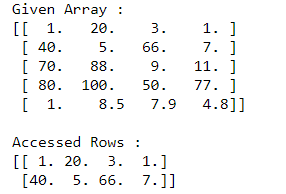In the above example, we access and print the First two rows of the 5X4 NumPy array.

Case 2: In 3-Dimensional arrays

Example 1: Accessing the Middle rows of 3-D NumPy array

## Python3

 `# Importing Numpy module ``import` `numpy as np`` ` `# Creating 3-D Numpy array``n_arr ``=` `np.array([[[``10``, ``25``, ``70``], [``30``, ``45``, ``55``], [``20``, ``45``, ``7``]], ``                  ``[[``50``, ``65``, ``8``], [``70``, ``85``, ``10``], [``11``, ``22``, ``33``]]])`` ` `print``(``"Given 3-D Array:"``)``print``(n_arr)`` ` `# Access the Middle rows of 3-D array``res_arr ``=` `n_arr[:,[``1``]]``print``(``"\nAccessed Rows :"``)``print``(res_arr)`

Output: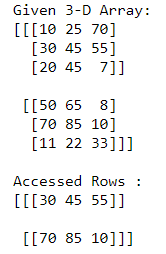In the above example, we access and print the Middle rows of the 3-D NumPy array.

Example 2: Accessing the First and Last rows of 3-D NumPy array

## Python3

 `# Importing Numpy module ``import` `numpy as np`` ` `# Creating 3-D Numpy array``n_arr ``=` `np.array([[[``10``, ``25``, ``70``], [``30``, ``45``, ``55``], [``20``, ``45``, ``7``]], ``                  ``[[``50``, ``65``, ``8``], [``70``, ``85``, ``10``], [``11``, ``22``, ``33``]],``                 ``[[``19``, ``69``, ``36``], [``1``, ``5``, ``24``], [``4``, ``20``, ``96``]]])`` ` ` ` `print``(``"Given 3-D Array:"``)``print``(n_arr)`` ` `# Access the First and Last rows of 3-D array``res_arr ``=` `n_arr[:,[``0``, ``2``]]``print``(``"\nAccessed Rows :"``)``print``(res_arr)`

Output: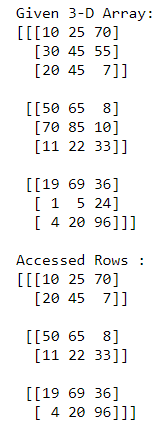In the above example, we access and print the First and Last rows of the 3-D NumPy array.

My Personal Notes arrow_drop_up